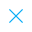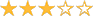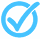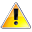Call Support +91-85588-96644

Keep me logged in
You can't leave Captcha Code empty
By submitting this form, you agree to the Terms & Privacy Policy.
ORTests given

OR# Linear Equations| By TCY

Questions

Time

Highest
score

Level

## ENGLISH

Language

Topics Covered:Simultaneous equations and graphsPair of Linear Equations in Two VariablesLinear Equations in Two VariablesSolving Pair of Linear Equations by Elimination MethodLinear Equation in 2 VariablesLinear Equations with One and Two VariablesLinear Equations in 2 VariablesSolving equationsGraphical Solution and Finding Areas of Right Triangles FormedMathematicsThis is a concept-building practice test and may not have exact structure as you would expect in the actual exam. Please exercise your discretion to attempt it or go to structured Featured Section.

Description:
Equations_1
Keywords:
•